# 8 4 Functions Transforming Quadratic Functions 8 4

• Slides: 38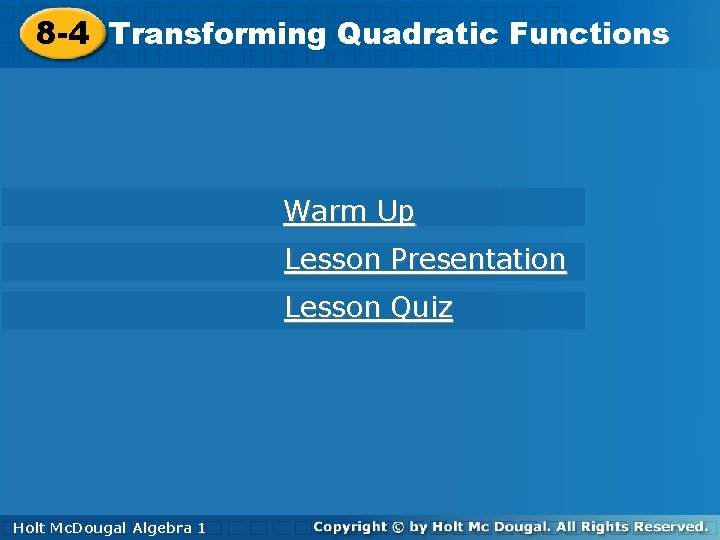8 -4 Functions Transforming Quadratic Functions 8 -4 Transforming Warm Up Lesson Presentation Lesson Quiz Holt Mc. Dougal Algebra 1 Algebra 11 Holt Mc. Dougal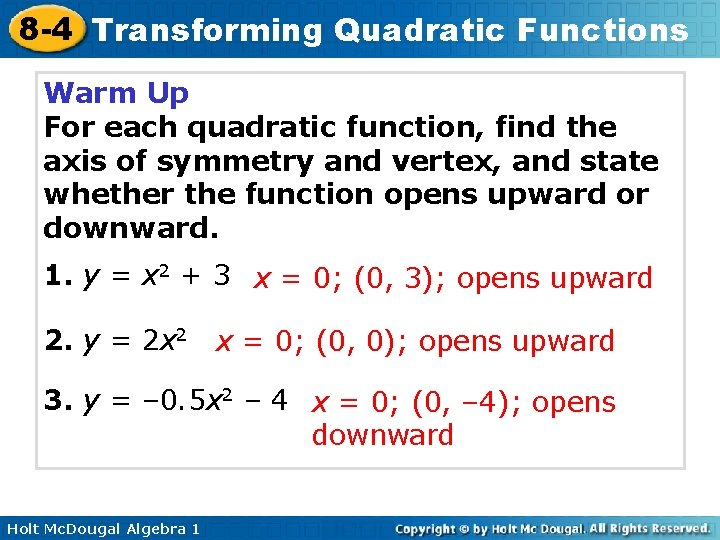8 -4 Transforming Quadratic Functions Warm Up For each quadratic function, find the axis of symmetry and vertex, and state whether the function opens upward or downward. 1. y = x 2 + 3 x = 0; (0, 3); opens upward 2. y = 2 x 2 x = 0; (0, 0); opens upward 3. y = – 0. 5 x 2 – 4 x = 0; (0, – 4); opens downward Holt Mc. Dougal Algebra 18 -4 Transforming Quadratic Functions Objective Graph and transform quadratic functions. Holt Mc. Dougal Algebra 18 -4 Transforming Quadratic Functions Remember! You saw in Lesson 5 -10 that the graphs of all linear functions are transformations of the linear parent function y = x. Holt Mc. Dougal Algebra 1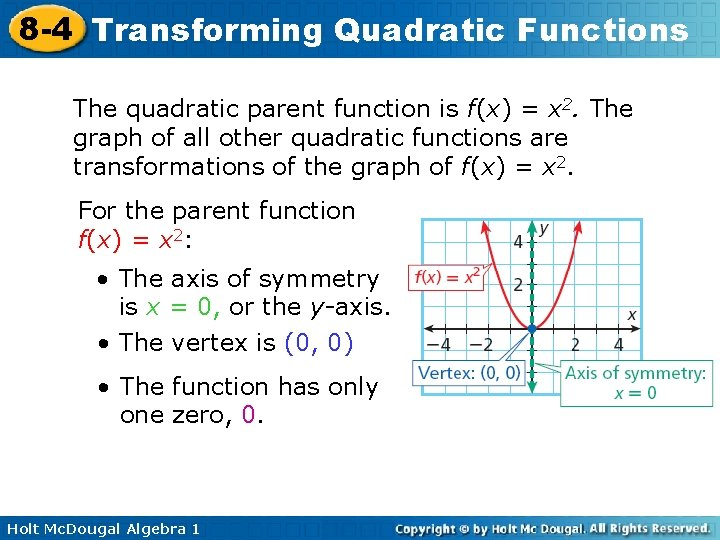8 -4 Transforming Quadratic Functions The quadratic parent function is f(x) = x 2. The graph of all other quadratic functions are transformations of the graph of f(x) = x 2. For the parent function f(x) = x 2: • The axis of symmetry is x = 0, or the y-axis. • The vertex is (0, 0) • The function has only one zero, 0. Holt Mc. Dougal Algebra 1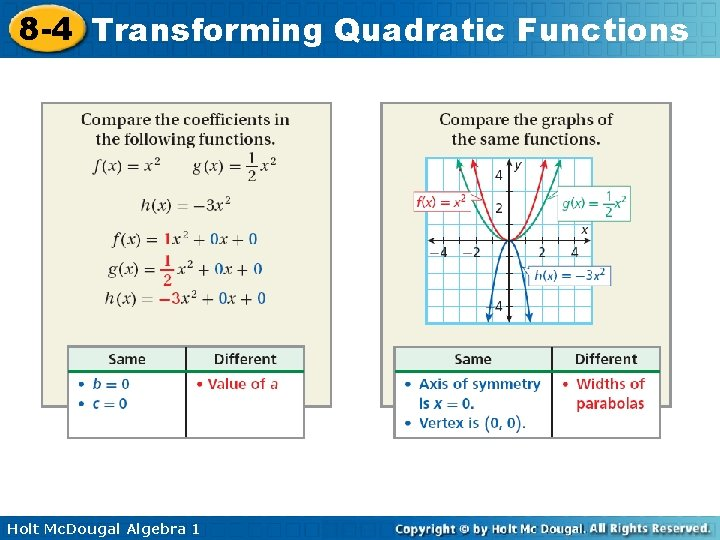8 -4 Transforming Quadratic Functions Holt Mc. Dougal Algebra 18 -4 Transforming Quadratic Functions The value of a in a quadratic function determines not only the direction a parabola opens, but also the width of the parabola. Holt Mc. Dougal Algebra 1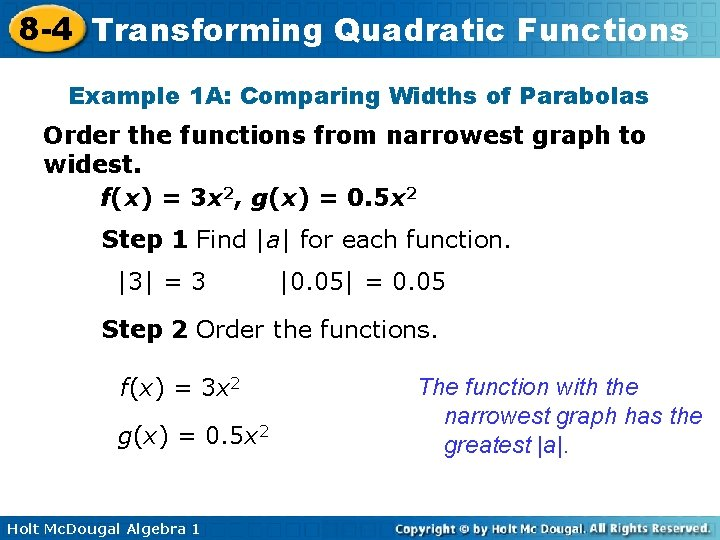8 -4 Transforming Quadratic Functions Example 1 A: Comparing Widths of Parabolas Order the functions from narrowest graph to widest. f(x) = 3 x 2, g(x) = 0. 5 x 2 Step 1 Find |a| for each function. |3| = 3 |0. 05| = 0. 05 Step 2 Order the functions. f(x) = 3 x 2 g(x) = 0. 5 x 2 Holt Mc. Dougal Algebra 1 The function with the narrowest graph has the greatest |a|.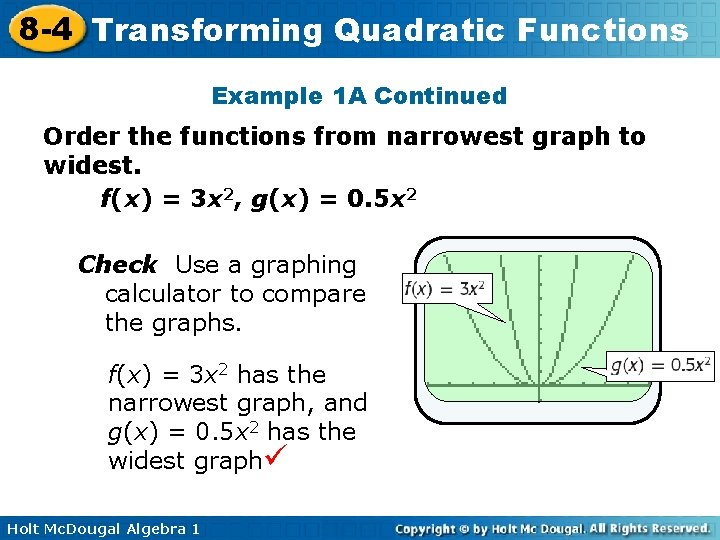8 -4 Transforming Quadratic Functions Example 1 A Continued Order the functions from narrowest graph to widest. f(x) = 3 x 2, g(x) = 0. 5 x 2 Check Use a graphing calculator to compare the graphs. f(x) = 3 x 2 has the narrowest graph, and g(x) = 0. 5 x 2 has the widest graph Holt Mc. Dougal Algebra 1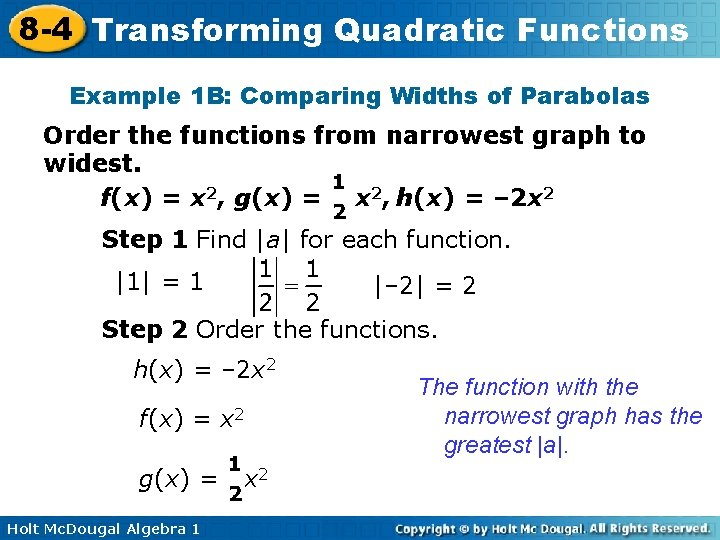8 -4 Transforming Quadratic Functions Example 1 B: Comparing Widths of Parabolas Order the functions from narrowest graph to widest. f(x) = x 2, g(x) = x 2, h(x) = – 2 x 2 Step 1 Find |a| for each function. |1| = 1 |– 2| = 2 Step 2 Order the functions. h(x) = – 2 x 2 f(x) = x 2 g(x) = Holt Mc. Dougal Algebra 1 x 2 The function with the narrowest graph has the greatest |a|.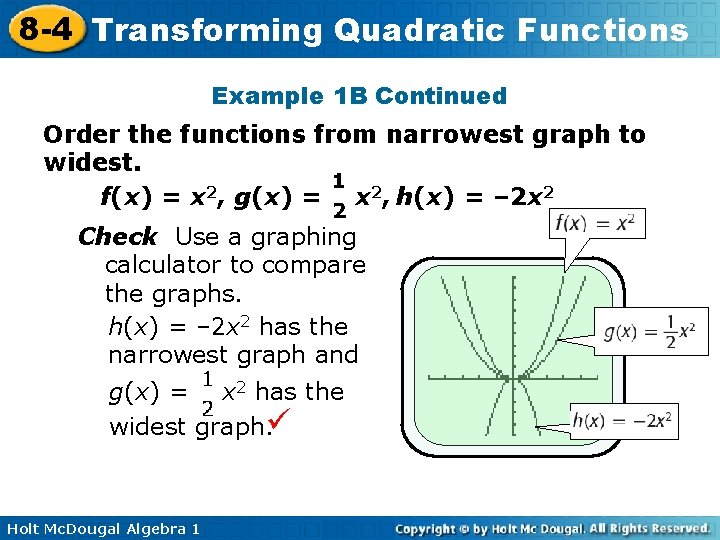8 -4 Transforming Quadratic Functions Example 1 B Continued Order the functions from narrowest graph to widest. f(x) = x 2, g(x) = x 2, h(x) = – 2 x 2 Check Use a graphing calculator to compare the graphs. h(x) = – 2 x 2 has the narrowest graph and g(x) = x 2 has the widest graph. Holt Mc. Dougal Algebra 18 -4 Transforming Quadratic Functions Check It Out! Example 1 a Order the functions from narrowest graph to widest. f(x) = –x 2, g(x) = x 2 Step 1 Find |a| for each function. |– 1| = 1 Step 2 Order the functions. f(x) = –x 2 g(x) = Holt Mc. Dougal Algebra 1 x 2 The function with the narrowest graph has the greatest |a|.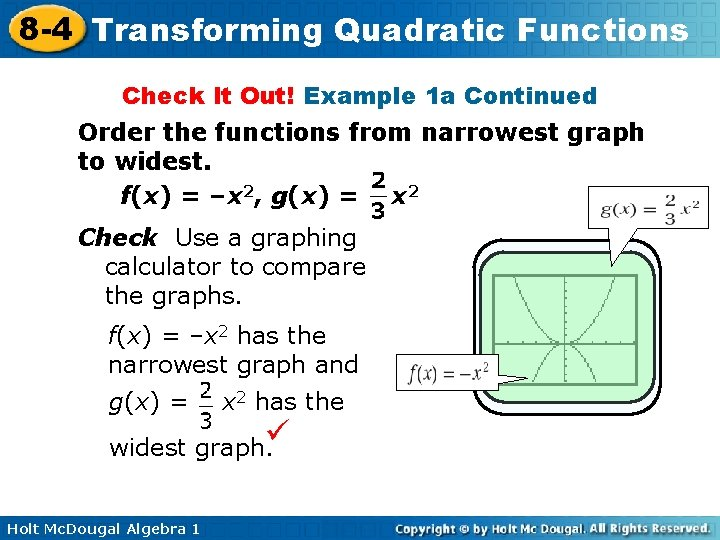8 -4 Transforming Quadratic Functions Check It Out! Example 1 a Continued Order the functions from narrowest graph to widest. f(x) = –x 2, g(x) = x 2 Check Use a graphing calculator to compare the graphs. f(x) = –x 2 has the narrowest graph and g(x) = x 2 has the widest graph. Holt Mc. Dougal Algebra 18 -4 Transforming Quadratic Functions Check It Out! Example 1 b Order the functions from narrowest graph to widest. f(x) = – 4 x 2, g(x) = 6 x 2, h(x) = 0. 2 x 2 Step 1 Find |a| for each function. |– 4| = 4 |6| = 6 |0. 2| = 0. 2 Step 2 Order the functions. g(x) = 6 x 2 f(x) = – 4 x 2 h(x) = 0. 2 x 2 Holt Mc. Dougal Algebra 1 The function with the narrowest graph has the greatest |a|.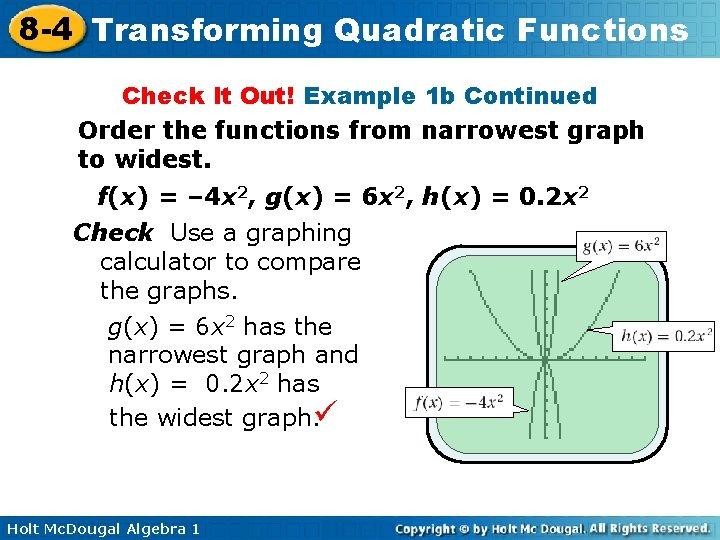8 -4 Transforming Quadratic Functions Check It Out! Example 1 b Continued Order the functions from narrowest graph to widest. f(x) = – 4 x 2, g(x) = 6 x 2, h(x) = 0. 2 x 2 Check Use a graphing calculator to compare the graphs. g(x) = 6 x 2 has the narrowest graph and h(x) = 0. 2 x 2 has the widest graph. Holt Mc. Dougal Algebra 1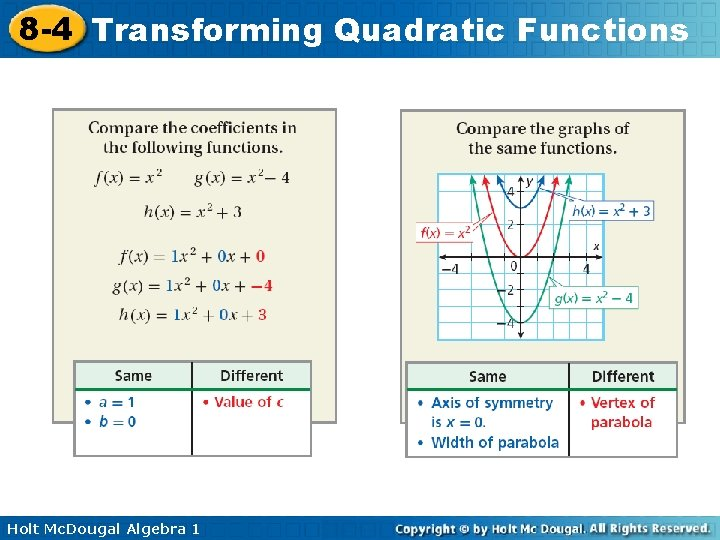8 -4 Transforming Quadratic Functions Holt Mc. Dougal Algebra 1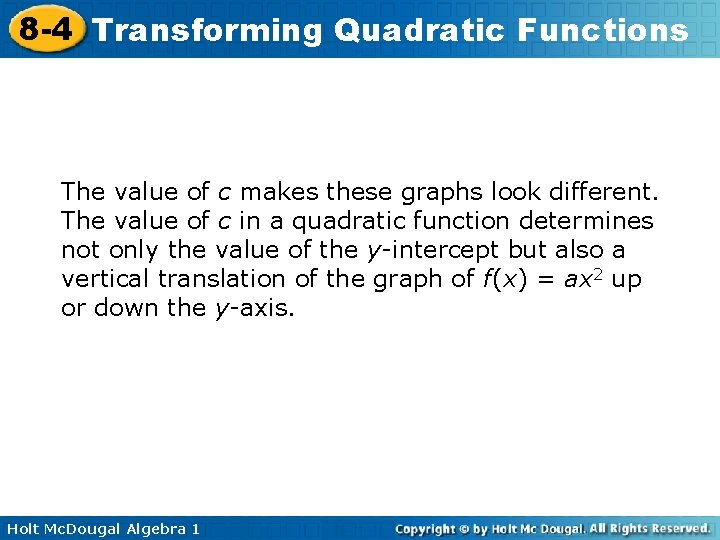8 -4 Transforming Quadratic Functions The value of c makes these graphs look different. The value of c in a quadratic function determines not only the value of the y-intercept but also a vertical translation of the graph of f(x) = ax 2 up or down the y-axis. Holt Mc. Dougal Algebra 18 -4 Transforming Quadratic Functions Holt Mc. Dougal Algebra 1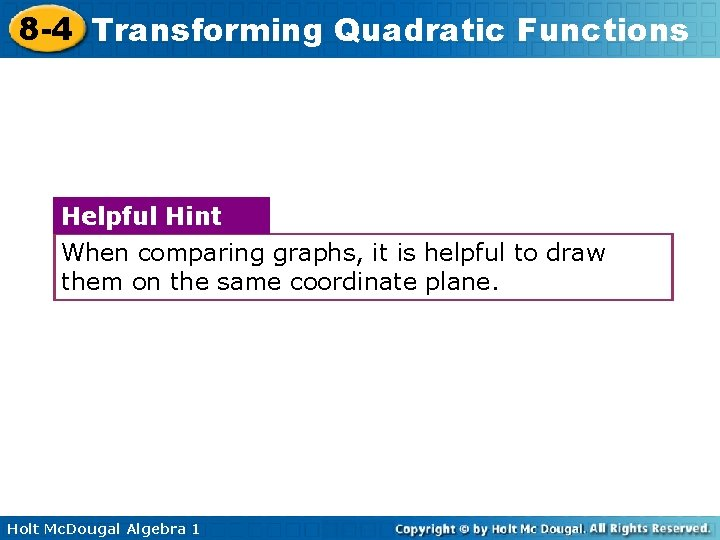8 -4 Transforming Quadratic Functions Helpful Hint When comparing graphs, it is helpful to draw them on the same coordinate plane. Holt Mc. Dougal Algebra 18 -4 Transforming Quadratic Functions Example 2 A: Comparing Graphs of Quadratic Functions Compare the graph of the function with the graph of f(x) = x 2. g(x) = x 2 + 3 Method 1 Compare the graphs. • The graph of g(x) = x 2 + 3 is wider than the graph of f(x) = x 2. • The graph of g(x) = x 2 + 3 opens downward and the graph of f(x) = x 2 opens upward. Holt Mc. Dougal Algebra 18 -4 Transforming Quadratic Functions Example 2 A Continued Compare the graph of the function with the graph of f(x) = x 2 g(x) = • x 2 + 3 The axis of symmetry is the same. • The vertex of f(x) = x 2 is (0, 0). The vertex of g(x) = x 2 + 3 is translated 3 units up to (0, 3). Holt Mc. Dougal Algebra 18 -4 Transforming Quadratic Functions Example 2 B: Comparing Graphs of Quadratic Functions Compare the graph of the function with the graph of f(x) = x 2 g(x) = 3 x 2 Method 2 Use the functions. • Since |3| > |1|, the graph of g(x) = 3 x 2 is narrower than the graph of f(x) = x 2. Since for both functions, the axis of symmetry is the same. • The vertex of f(x) = x 2 is (0, 0). The vertex of g(x) = 3 x 2 is also (0, 0). • • Both graphs open upward. Holt Mc. Dougal Algebra 18 -4 Transforming Quadratic Functions Example 2 B Continued Compare the graph of the function with the graph of f(x) = x 2 g(x) = 3 x 2 Check Use a graph to verify all comparisons. Holt Mc. Dougal Algebra 18 -4 Transforming Quadratic Functions Check It Out! Example 2 a Compare the graph of each the graph of f(x) = x 2. g(x) = –x 2 – 4 Method 1 Compare the graphs. • The graph of g(x) = –x 2 – 4 opens downward and the graph of f(x) = x 2 opens upward. • The axis of symmetry is the same. • The vertex of f(x) = x 2 is (0, 0). The vertex of g(x) = –x 2 – 4 is translated 4 units down to (0, – 4). Holt Mc. Dougal Algebra 1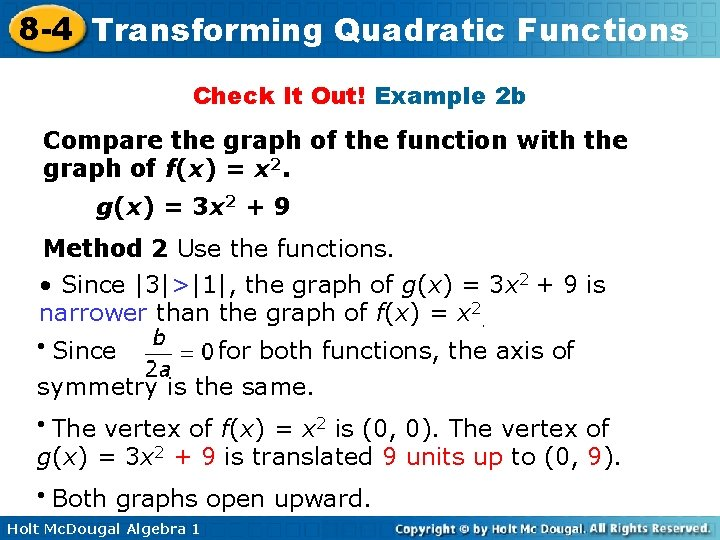8 -4 Transforming Quadratic Functions Check It Out! Example 2 b Compare the graph of the function with the graph of f(x) = x 2. g(x) = 3 x 2 + 9 Method 2 Use the functions. • Since |3|>|1|, the graph of g(x) = 3 x 2 + 9 is narrower than the graph of f(x) = x 2. Since for both functions, the axis of symmetry is the same. • The vertex of f(x) = x 2 is (0, 0). The vertex of g(x) = 3 x 2 + 9 is translated 9 units up to (0, 9). • • Both graphs open upward. Holt Mc. Dougal Algebra 1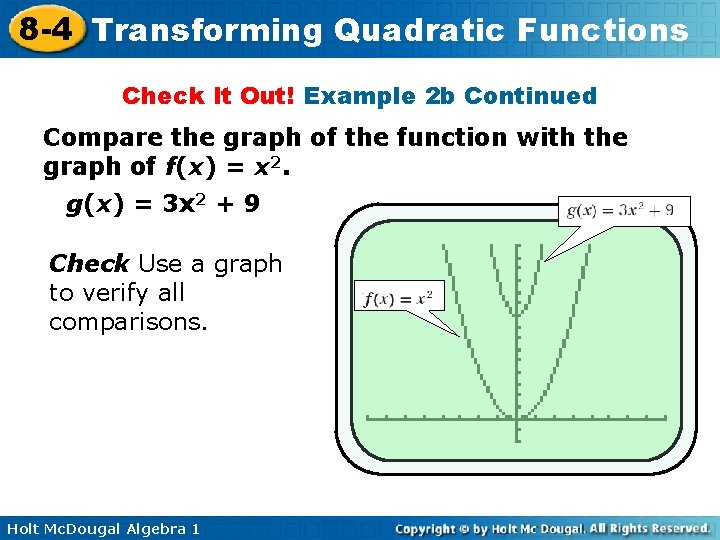8 -4 Transforming Quadratic Functions Check It Out! Example 2 b Continued Compare the graph of the function with the graph of f(x) = x 2. g(x) = 3 x 2 + 9 Check Use a graph to verify all comparisons. Holt Mc. Dougal Algebra 1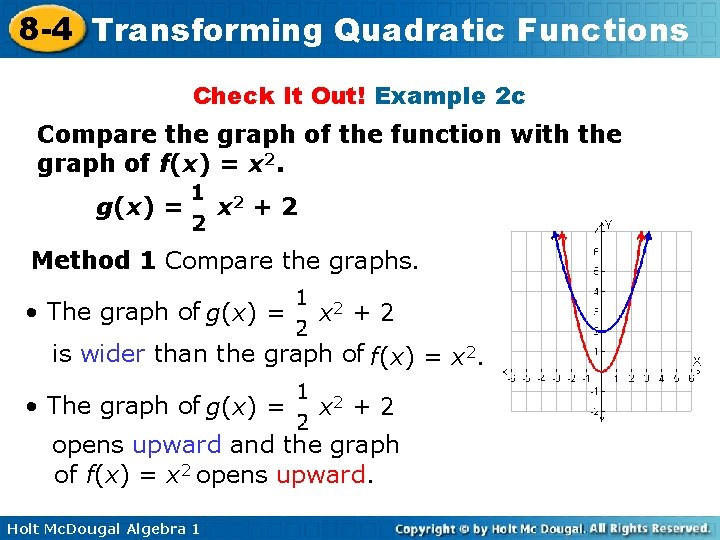8 -4 Transforming Quadratic Functions Check It Out! Example 2 c Compare the graph of the function with the graph of f(x) = x 2. g(x) = x 2 + 2 Method 1 Compare the graphs. • The graph of g(x) = x 2 + 2 is wider than the graph of f(x) = x 2. • The graph of g(x) = x 2 + 2 opens upward and the graph of f(x) = x 2 opens upward. Holt Mc. Dougal Algebra 1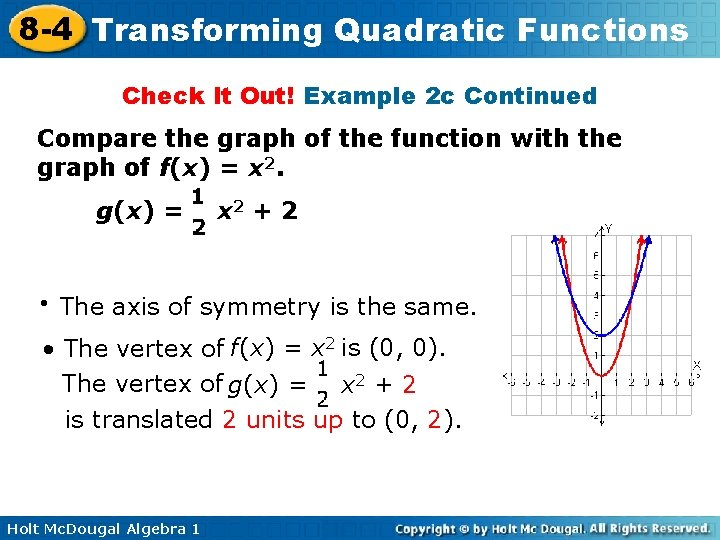8 -4 Transforming Quadratic Functions Check It Out! Example 2 c Continued Compare the graph of the function with the graph of f(x) = x 2. g(x) = • x 2 + 2 The axis of symmetry is the same. • The vertex of f(x) = x 2 is (0, 0). The vertex of g(x) = x 2 + 2 is translated 2 units up to (0, 2). Holt Mc. Dougal Algebra 18 -4 Transforming Quadratic Functions The quadratic function h(t) = – 16 t 2 + c can be used to approximate the height h in feet above the ground of a falling object t seconds after it is dropped from a height of c feet. This model is used only to approximate the height of falling objects because it does not account for air resistance, wind, and other real-world factors. Holt Mc. Dougal Algebra 18 -4 Transforming Quadratic Functions Example 3: Application Two identical softballs are dropped. The first is dropped from a height of 400 feet and the second is dropped from a height of 324 feet. a. Write the two height functions and compare their graphs. Step 1 Write the height functions. The y-intercept c represents the original height. h 1(t) = – 16 t 2 + 400 Dropped from 400 feet. h 2(t) = – 16 t 2 + 324 Dropped from 324 feet. Holt Mc. Dougal Algebra 1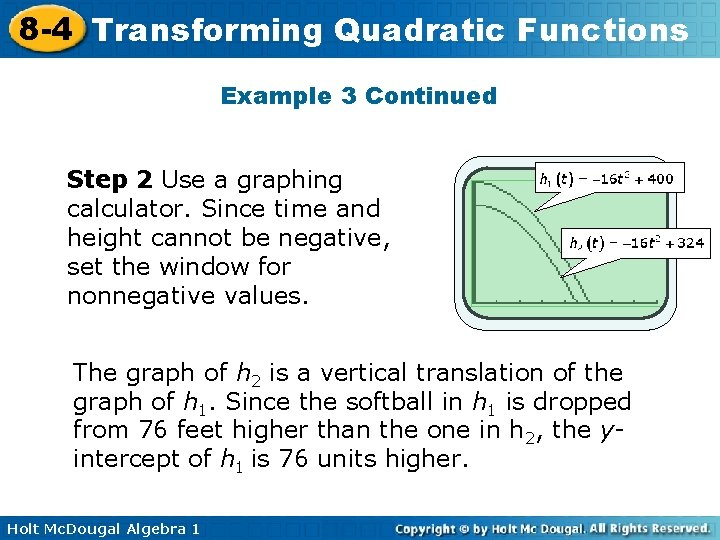8 -4 Transforming Quadratic Functions Example 3 Continued Step 2 Use a graphing calculator. Since time and height cannot be negative, set the window for nonnegative values. The graph of h 2 is a vertical translation of the graph of h 1. Since the softball in h 1 is dropped from 76 feet higher than the one in h 2, the yintercept of h 1 is 76 units higher. Holt Mc. Dougal Algebra 1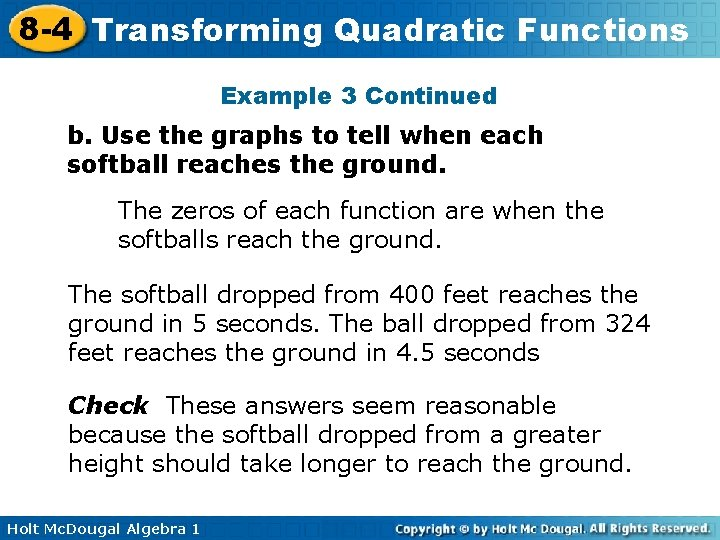8 -4 Transforming Quadratic Functions Example 3 Continued b. Use the graphs to tell when each softball reaches the ground. The zeros of each function are when the softballs reach the ground. The softball dropped from 400 feet reaches the ground in 5 seconds. The ball dropped from 324 feet reaches the ground in 4. 5 seconds Check These answers seem reasonable because the softball dropped from a greater height should take longer to reach the ground. Holt Mc. Dougal Algebra 18 -4 Transforming Quadratic Functions Caution! Remember that the graphs shown here represent the height of the objects over time, not the paths of the objects. Holt Mc. Dougal Algebra 1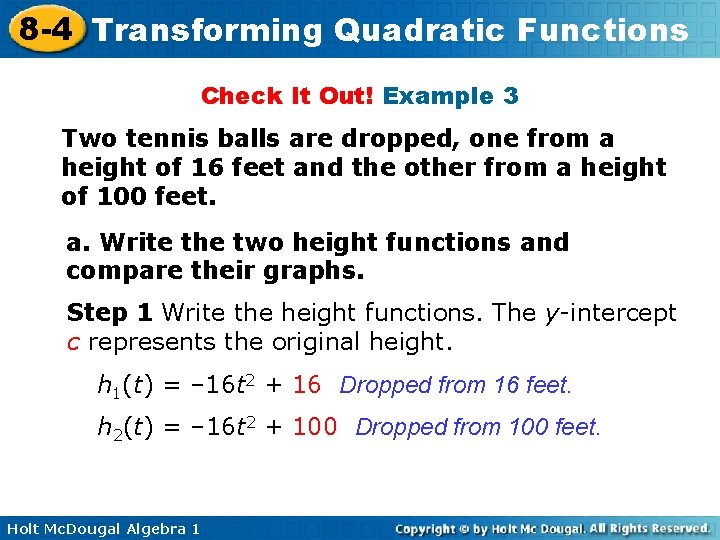8 -4 Transforming Quadratic Functions Check It Out! Example 3 Two tennis balls are dropped, one from a height of 16 feet and the other from a height of 100 feet. a. Write the two height functions and compare their graphs. Step 1 Write the height functions. The y-intercept c represents the original height. h 1(t) = – 16 t 2 + 16 Dropped from 16 feet. h 2(t) = – 16 t 2 + 100 Dropped from 100 feet. Holt Mc. Dougal Algebra 18 -4 Transforming Quadratic Functions Check It Out! Example 3 Continued Step 2 Use a graphing calculator. Since time and height cannot be negative, set the window for nonnegative values. The graph of h 2 is a vertical translation of the graph of h 1. Since the ball in h 2 is dropped from 84 feet higher than the one in h 1, the y-intercept of h 2 is 84 units higher. Holt Mc. Dougal Algebra 1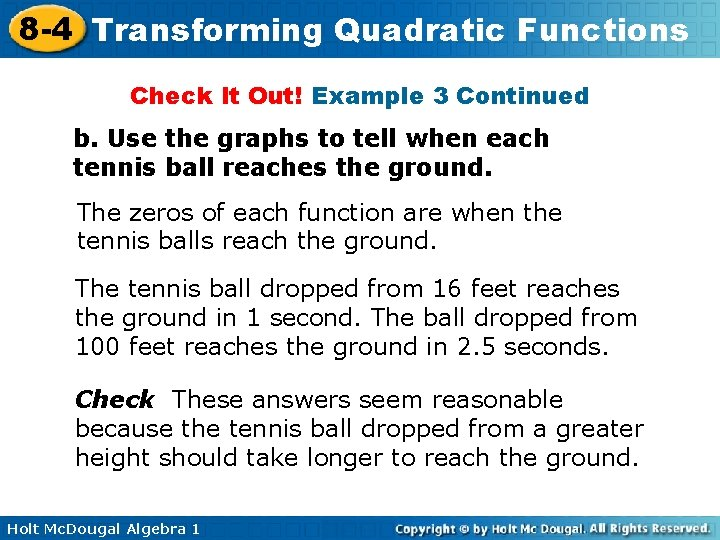8 -4 Transforming Quadratic Functions Check It Out! Example 3 Continued b. Use the graphs to tell when each tennis ball reaches the ground. The zeros of each function are when the tennis balls reach the ground. The tennis ball dropped from 16 feet reaches the ground in 1 second. The ball dropped from 100 feet reaches the ground in 2. 5 seconds. Check These answers seem reasonable because the tennis ball dropped from a greater height should take longer to reach the ground. Holt Mc. Dougal Algebra 1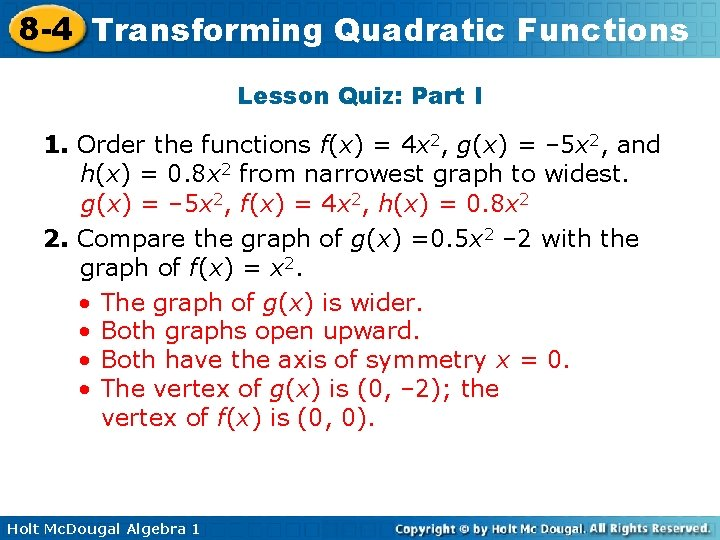8 -4 Transforming Quadratic Functions Lesson Quiz: Part I 1. Order the functions f(x) = 4 x 2, g(x) = – 5 x 2, and h(x) = 0. 8 x 2 from narrowest graph to widest. g(x) = – 5 x 2, f(x) = 4 x 2, h(x) = 0. 8 x 2 2. Compare the graph of g(x) =0. 5 x 2 – 2 with the graph of f(x) = x 2. • The graph of g(x) is wider. • Both graphs open upward. • Both have the axis of symmetry x = 0. • The vertex of g(x) is (0, – 2); the vertex of f(x) is (0, 0). Holt Mc. Dougal Algebra 1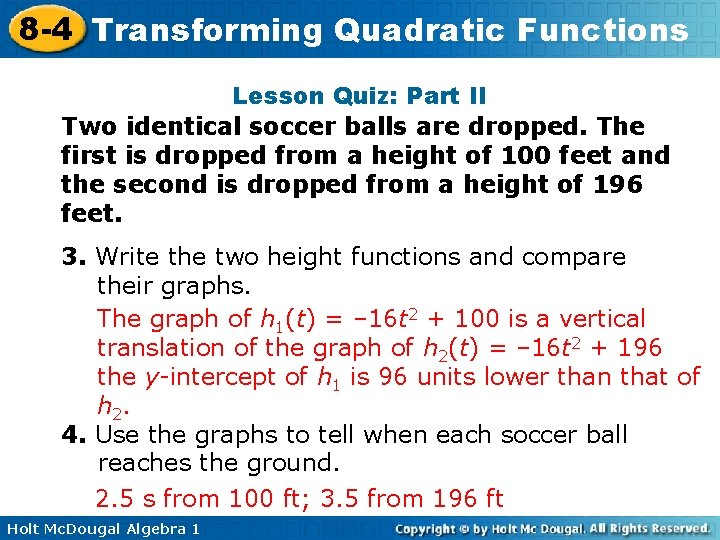8 -4 Transforming Quadratic Functions Lesson Quiz: Part II Two identical soccer balls are dropped. The first is dropped from a height of 100 feet and the second is dropped from a height of 196 feet. 3. Write the two height functions and compare their graphs. The graph of h 1(t) = – 16 t 2 + 100 is a vertical translation of the graph of h 2(t) = – 16 t 2 + 196 the y-intercept of h 1 is 96 units lower than that of h 2. 4. Use the graphs to tell when each soccer ball reaches the ground. 2. 5 s from 100 ft; 3. 5 from 196 ft Holt Mc. Dougal Algebra 1